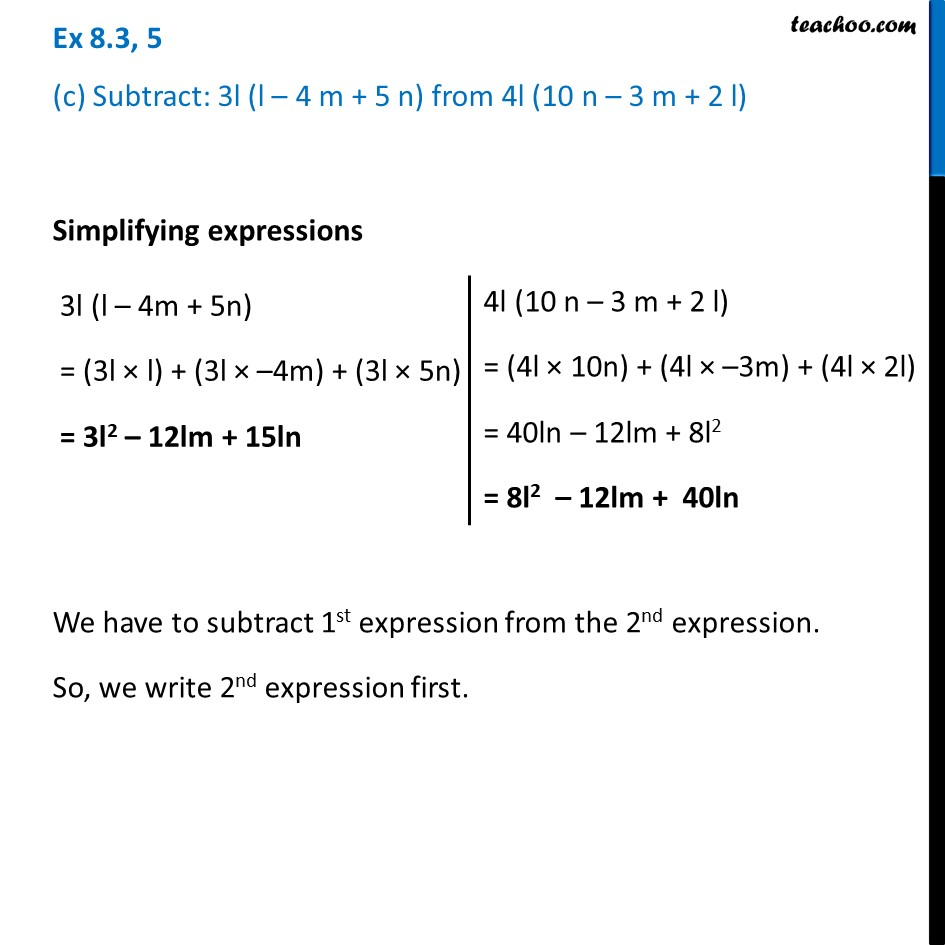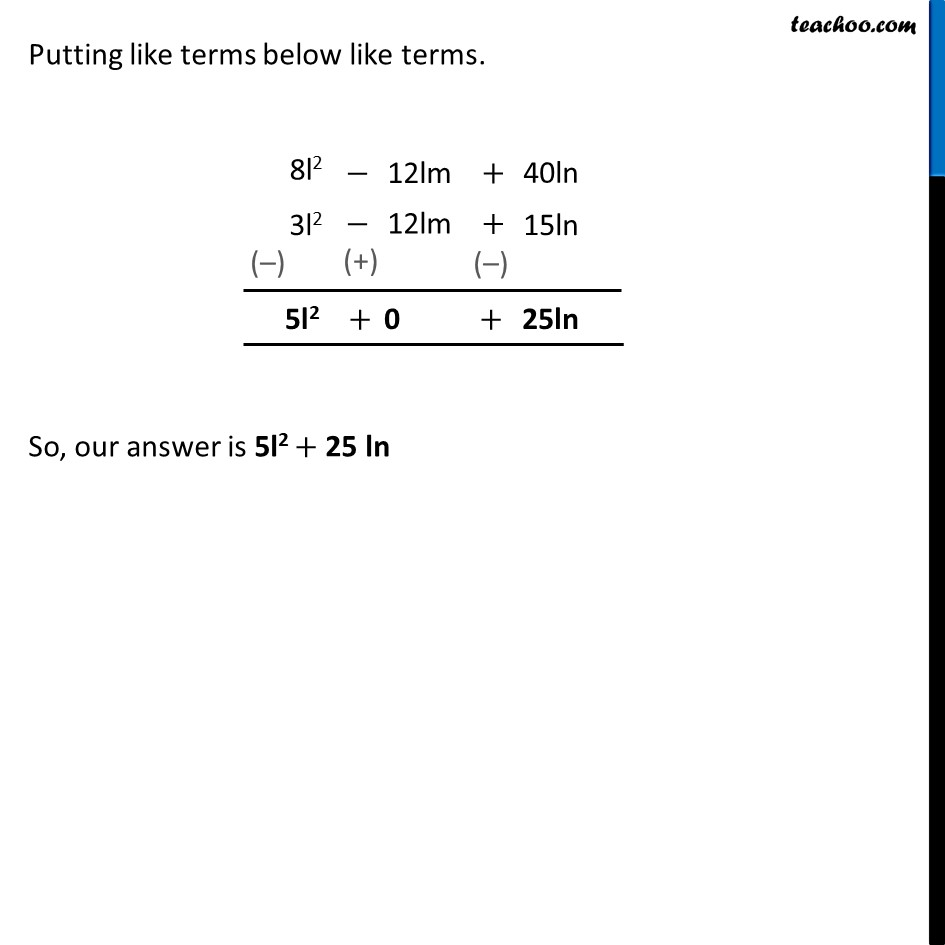Ex 8.3

Chapter 8 Class 8 Algebraic Expressions and Identities
Serial order wiseLearn in your speed, with individual attention - Teachoo Maths 1-on-1 Class

### Transcript

Ex 8.3, 5 (c) Subtract: 3l (l – 4 m + 5 n) from 4l (10 n – 3 m + 2 l)3l (l – 4m + 5n) = (3l × l) + (3l × –4m) + (3l × 5n) = 3l2 – 12lm + 15ln 4l (10 n – 3 m + 2 l) = (4l × 10n) + (4l × –3m) + (4l × 2l) = 40ln – 12lm + 8l2 = 8l2 – 12lm + 40ln Simplifying expressions We have to subtract 1st expression from the 2nd expression. So, we write 2nd expression first. Putting like terms below like terms. So, our answer is 5l2 + 25 ln